# Domain & Range Of A Rational Function (3 Key Ideas)

When we work with rational functions, we often want to find the domain and range.  This helps us to graph the function, including any horizontal and vertical asymptotes.

So, what is the domain and range of a rational function?  The domain of a rational function is the set of all real numbers except those that cause a zero denominator. The range of a rational function is the set of possible output values, which is often found by analyzing the graph or finding the domain of the inverse of the function.

Of course, we must be comfortable with various factoring methods (including difference of squares, perfect square trinomials, etc.) to be able to find the zeros of the denominator of a rational function.

In this article, we’ll talk about how to find the domain and range of a rational function, both with algebra and by looking at graphs.

Let’s get started.

## Domain & Range Of A Rational Function

First, it is important to define the key terms.  Remember that a rational function is a quotient of two polynomials.

That is, a rational function R(x) has the form P(x) / Q(x), where P(x) and Q(x) are polynomials.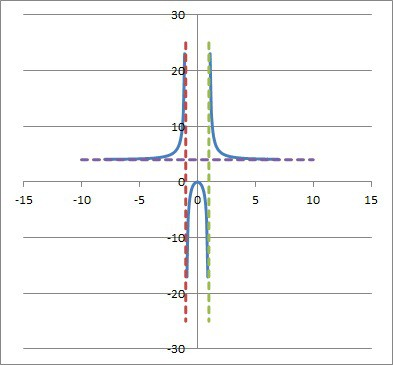A rational function is a quotient of polynomials, and it can have both horizontal and vertical asymptotes (sometimes more than one of each).

A polynomial is a sum of terms of the form anxn, where an is a coefficient and xn is a variable raised to the nth power.  For example, the following are polynomials:

• 5
• 2x + 5
• x2 + 5
• x2 + 3x + 5
• x3 – 4x2 + 2x – 7
• …etc.

A rational function is just a quotient of polynomials like the ones above.  For example, the following are rational functions:

• 5 / (x + 1)
• (x – 4) / (x2 – 9)
• (x2 + 2x – 5) / (x3 – 4x2 + 3x – 8)
• … etc.

Now that we know what a rational function is, we can find its domain and range.

In simple terms:

• The domain of a rational function is the set of inputs (x-values) that do not cause a zero denominator.  For example, the domain of f(x) = 1/x is all real numbers except x = 0, often denoted as R – {0}.
• The range of a rational function is the set of all possible outputs (y-values).  For example, the range of g(x) = 1/x2 is all positive real numbers, often denoted at R+.

The best way to analyze a rational function is to find the domain by checking for zero denominators (to find the domain) and then finding the possible set of outputs (to find the range).

Graphing can also help with both of these steps.

Let’s take a look at finding the domain of a rational function first, and then we’ll look at how to find the range.

### How To Find The Domain Of A Rational Function

To find the domain of a rational function, we need to find the inputs (x-values) that make the denominator zero and exclude them from the domain.

So, let’s say that our rational function is f(x) = P(x) / Q(x), where P(x) and Q(x) are polynomials.  Here are the steps to find the domain:

1. First, take the denominator polynomial and set it equal to zero; that is, Q(x) = 0.
2. Next, factor Q(x) completely, using factoring methods as necessary (such as difference of squares, factoring by grouping, perfect square trinomials, etc.)
3. Then, after factoring Q(x) completely, write out the solutions to the equation Q(x) = 0.
4. Finally, write the domain as the real numbers excluding the solutions in step 3.

Let’s look at some examples to see how this works.

#### Example 1: Find The Domain Of A Rational Function

Let’s say we have the rational function f(x) = 1 / x.

You can see a graph of the function below.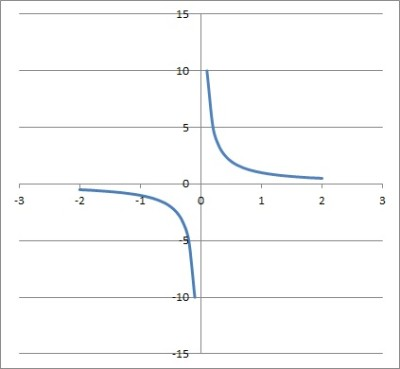This is the graph of the rational function f(x) = 1/x. This is also called the reciprocal function. It is its own inverse, and has a horizontal asymptote at x = 0 and a vertical asymptote at y = 0.

The denominator is the polynomial Q(x) = x.

Our first step is to set Q(x) equal to zero: this gives us the equation x = 0.

Our second step is to factor Q(x) completely.  This is already done, since x is irreducible.

Our third step is to write out the solutions to Q(x) = 0.  This gives the single solution x = 0.

Our fourth step is to write the domain of f(x) as R – {0}.

Note that we could also write the domain as the union of two sets: (-∞, 0)u(0, ∞).]

#### Example 2: Find The Domain Of A Rational Function

Let’s say we have the rational function f(x) = (x + 2) / (x2 – 9).

You can see a graph of the function below.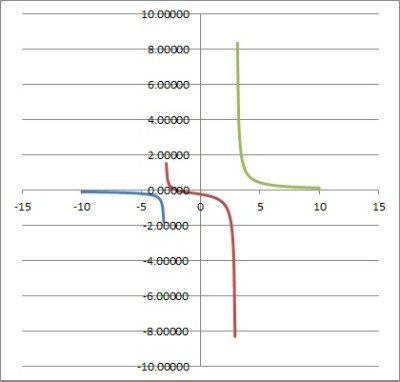The graph of the rational function f(x) = (x + 2) / (x2 – 9) has vertical asymptotes at x = -3 and x = 3, with a horizontal asymptote at y = 0.

The denominator is the polynomial Q(x) = x2 – 9.

Our first step is to set Q(x) equal to zero: this gives us the equation x2 – 9 = 0.

Our second step is to factor Q(x) completely.  We notice that x2 – 9 is a difference of squares, which factors as (x + 3)(x – 3).

Our third step is to write out the solutions to Q(x) = 0.  For (x + 3)(x – 3) = 0, the two solutions are x = -3 and x = 3.

Our fourth step is to write the domain of f(x) as R – {-3, 3}.

Note that we could also write the domain as the union of three sets: (-∞, -3)u(-3, 3)u(3, ∞).

#### Example 3: Find The Domain Of A Rational Function

Let’s say we have the rational function f(x) = (x2 + 3) / (x3 + 3x2 + 2x).

You can see a graph of the function below.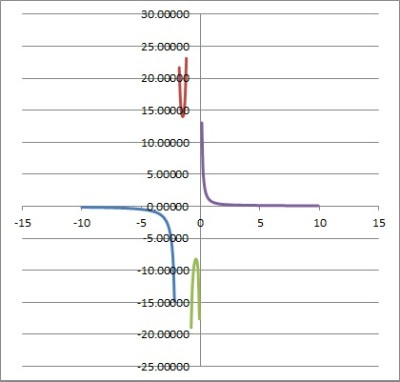The graph of the rational function f(x) = (x2 + 3) / (x3 + 3x2 + 2x), which has vertical asymptotes at x = -2, x = -1, and x = 0, as well as a horizontal asymptote at y = 0.

The denominator is the polynomial Q(x) = x3 + 3x2 + 2x.

Our first step is to set Q(x) equal to zero: this gives us the equation x3 + 3x2 + 2x = 0.

Our second step is to factor Q(x) completely.  We notice that we can factor out a GCF (greatest common factor) of x, and then go from there:

• x3 + 3x2 + 2x = 0  [Q(x) = 0]
• x(x2 + 3x + 2) = 0  [factor out a GCF of x]
• x(x+ 1)(x + 2) = 0

Our third step is to write out the solutions to Q(x) = 0.  For x(x + 1)(x + 2) = 0, the three solutions are x = 0, x = -1, and x = -2.

Our fourth step is to write the domain of f(x) as R – {-2, -1, 0}.

Note that we could also write the domain as the union of four sets: (-∞, -2)u(-2, -1)u(-1, 0)u(0, ∞).

#### Example 4: Find The Domain Of A Rational Function

Let’s say we have the rational function f(x) = (x3 + 7x + 1) / (x4 + 2x3 – x2 – 2x).

You can see a graph of the function below.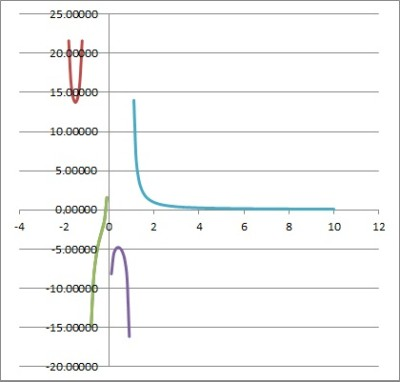The graph of the rational function f(x) = (x3 + 7x + 1) / (x4 + 2x3 – x2 – 2x), which has vertical asymptotes at x = -2, -1, 0, and 1, with a horizontal asymptote at y = 0.

The denominator is the polynomial Q(x) = x4 + 2x3 – x2 – 2x.

Our first step is to set Q(x) equal to zero: this gives us the equation x4 + 2x3 – x2 – 2x = 0.

Our second step is to factor Q(x) completely.  We will try to factor by grouping, looking at pairs of terms:

• x4 + 2x3 – x2 – 2x = 0  [Q(x) = 0]
• (x4 + 2x3) – (x2 + 2x) = 0  [group pairs of terms to help factor]
• x3(x + 2) – x(x + 2) = 0  [factor out a GCF from each pair]
• (x3 – x)(x + 2) = 0
• x(x2 – 1)(x + 2) = 0 [factor out a GCF of x from x3 – x2]
• x(x + 1)(x – 1)(x + 2) = 0  [factor x2 – 1 as a difference of squares]

Our third step is to write out the solutions to Q(x) = 0.  For x(x + 1)(x – 1)(x + 2) = 0, the four solutions are x = -2, x = -1, x = 0, and x = 1.

Our fourth step is to write the domain of f(x) as R – {-2, -1, 0, 1}.

Note that we could also write the domain as the union of five sets: (-∞, -2)u(-2, -1)u(-1, 0)u(0, 1)u(1, ∞).

### How To Find The Range Of A Rational Function

To find the range of a rational function, there are a few approaches we can take.

One method to find the inverse function and take its domain, which is the range of the original function.

Another method is to graph the function and use it to help us find the range (by using horizontal asymptotes).

Let’s look at some examples to see how this works.

#### Example 1: Find The Range Of A Rational Function

Let’s say we have the rational function f(x) = 1/x.

Since this function is invertible on the domain (x not zero), we can find the inverse and use the fact that the range of a function f(x) is the domain of its inverse f-1(x).

So, we have the function f(x) = 1/x.  We use the standard steps to find the inverse of a function:

• f(x) = 1/x  [original function]
• y = 1/x  [replace f(x) by y]
• x = 1/y  [switch the x and y variables]
• xy = 1  [multiply by y on both sides]
• y = 1/x  [divide by x on both sides]
• f-1(x) = 1/x  [replace y by f-1(x)]

So, our inverse function is f-1(x) = 1/x.  We can see that this rational function has a denominator of x, which is zero when x = 0.

So, the domain of f-1(x) is R – {0}.  That means that the range of f(x) is R – {0}.  We can verify this with the graph of the function, shown below.The graph of the rational function f(x) = 1/x, which has a domain of x not equal to zero and a range of y not equal to zero.

#### Example 2: Find The Range Of A Rational Function

Let’s say we have the rational function f(x) = 1 / (x3 – 8).

Since this function is invertible on the domain (x not equal to 2), we can find the inverse and use the fact that the range of a function f(x) is the domain of its inverse f-1(x).

So, we have the function f(x) = 1 / (x3 – 8).  We use the standard steps to find the inverse of a function:

• f(x) = 1 / (x3 – 8)  [original function]
• y = 1 / (x3 – 8)  [replace f(x) by y]
• x = 1/(y3 – 8)    [switch the x and y variables]
• x(y3 – 8) = 1  [multiply by y3 – 8 on both sides]
• y3 – 8 = 1/x  [divide by x on both sides]
• y3 = (1/x) + 8)  [add 8 to both sides]
• y3 = (8x + 1)/x  [common denominator of 8]
• y = 3√((8x + 1)/x)  [take cube root of both sides]

So, our inverse function is f-1(x) = 3√((8x + 1)/8).  Note that the denominator of the fraction in the radicand cannot be zero.  So, x is not equal to zero.

Note that we can have a negative radicand here, since we are taking a cube root, rather than a square root.

So, the domain of f-1(x) is R – {0}.  That means that the range of f(x) is R – {0}.  We can verify this with the graph of the function, shown below.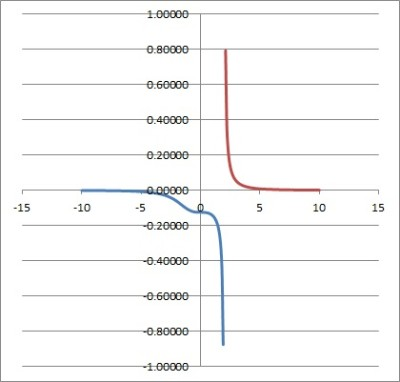This is the graph of the rational function f(x) = 1 / (x3 – 8), which has a vertical asymptote at x = 2 and a horizontal asymptote at y = 0.

#### Example 3: Find The Range Of A Rational Function

Let’s say we have the rational function f(x) = x / (x – 1).

Since this function is invertible on the domain (x not equal to 1), we can find the inverse and use the fact that the range of a function f(x) is the domain of its inverse f-1(x).

So, we have the function f(x) = x / (x – 1).  We use the standard steps to find the inverse of a function:

• f(x) = x / (x – 1)  [original function]
• y = x / (x – 1)  [replace f(x) by y]
• x = y / (y – 1)  [switch the x and y variables]
• x(y– 1) = y  [multiply by y – 1 on both sides]
• xy – x = y  [distribute through parentheses]
• xy – y = x  [isolate y on the left side]
• y(x – 1) = x  [factor out y]
• y = x / (x – 1)  [divide by x – 1 on both sides]

So, our inverse function is f-1(x) = x / (x – 1).  The denominator x – 1 cannot equal zero, so the domain of f-1(x) is R – {1}.

That means that the range of f(x) is R – {1}.  We can verify this with the graph of the function, shown below.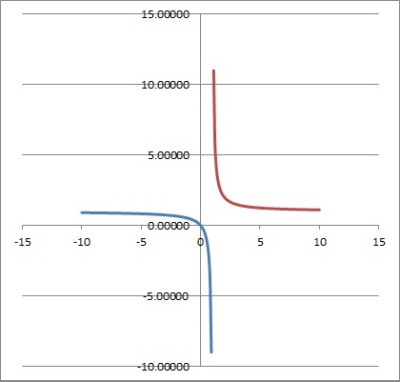This is the graph of the rational function f(x) = x / (x – 1), which has a vertical asymptote at x = 1 and a horizontal asymptote at y = 1.

## Conclusion

Now you know how to find both the domain and range of a rational function.  You also know how to look at the graphs to make sense of whether your solution makes sense.

You can learn how to find the domain and range of a polynomial here.